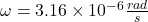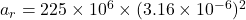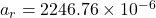## An undiscovered planet, many lightyears from Earth, has one moon in a periodic orbit. This moon takes 2010 × 103 seconds (about 23 days) on

Question

An undiscovered planet, many lightyears from Earth, has one moon in a periodic orbit. This moon takes 2010 × 103 seconds (about 23 days) on average to complete one nearly circular revolution around the unnamed planet. If the distance from the center of the moon to the surface of the planet is 225.0 × 106 m and the planet has a radius of 3.80 × 106 m, calculate the moon’s radial acceleration a c .

in progress 0
5 months 2021-08-13T09:50:28+00:00 1 Answers 0 views 0

Radial acceleration of moon is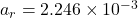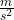Explanation:

Given :

Time period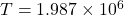sec

Distance from center of moon to planet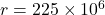m

From the equation of radial acceleration,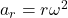Where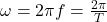So